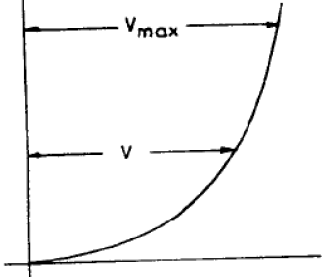Courses

# Boundary Layer Theory, Laminar Flow - MCQ Test 2

## 30 Questions MCQ Test GATE Mechanical (ME) 2022 Mock Test Series | Boundary Layer Theory, Laminar Flow - MCQ Test 2

Description
This mock test of Boundary Layer Theory, Laminar Flow - MCQ Test 2 for Mechanical Engineering helps you for every Mechanical Engineering entrance exam. This contains 30 Multiple Choice Questions for Mechanical Engineering Boundary Layer Theory, Laminar Flow - MCQ Test 2 (mcq) to study with solutions a complete question bank. The solved questions answers in this Boundary Layer Theory, Laminar Flow - MCQ Test 2 quiz give you a good mix of easy questions and tough questions. Mechanical Engineering students definitely take this Boundary Layer Theory, Laminar Flow - MCQ Test 2 exercise for a better result in the exam. You can find other Boundary Layer Theory, Laminar Flow - MCQ Test 2 extra questions, long questions & short questions for Mechanical Engineering on EduRev as well by searching above.
QUESTION: 1

Solution:

Ans. (c)

QUESTION: 2

### The MINIMUM value of friction factor ‘f’ that can occur in laminar flow through a circular pipe is:

Solution:

Ans. (b) Friction Factor,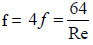Where Max. Re = 2000.

QUESTION: 3

### The integrated drag force (in N) on the plate, between p – s, is:

Solution:

Ans. (c) By momentum equation, we can find drag force.

QUESTION: 4

In the boundary layer, the flow is:

Solution:

Ans. (a)

QUESTION: 5

In the region of the boundary layer nearest to the wall where velocityis not equal to zero, the viscous forces are:

Solution:

Ans. (c) Reynold’s number = Inertia force / Viscous force and it is more than one therefore the viscous forces are less than  inertial forces.

QUESTION: 6

The hydrodynamic boundary layer thickness is defined as the distance from the surface where the

Solution:

Ans. (d)

QUESTION: 7

Assertion (A): The 'dimples' on a golf ball are intentionally provided.

Reason (R): A turbulent boundary layer, since it has more momentumthan a laminar boundary layer, can better resist an adverse pressure gradient.

Solution:

Ans. (a)

QUESTION: 8

During the flow over a circular cylinder, the drag coefficient dropssignificantly at a critical Reynolds number of 2 × 105. This is due to

Solution:

Ans. (d) The drag co-efficient remains practically constant unit a Reynold’s number of 2 × 105 is reached. At this stage the Cd drops steeply by a factor of 5.
This is due to the fact that the laminar boundary layer turns turbulent and stays unseparated over a longer distance, then reducing the wake considerably.

QUESTION: 9

What is the commonly used boundary layer control method to prevent separation?

Solution:

Ans. (d) Following are some of the methods generally adopted to retard separation:
1. Streamlining the body shape.
2. Tripping the boundary layer from laminar to turbulent by provision roughness.
3. Sucking the retarded flow.
4. Injecting high velocity fluid in the boundary  layer.
5. Providing slots near the leading edge.
6. Guidance of flow in a confined passage.
7. Providing a rotating cylinder near the leading edge.
8. Energizing the flow by introducing optimum amount of swirl in the incoming
flow.

QUESTION: 10

Prandtl number of a flowing fluid greater than unity indicates that hydrodynamic boundary layer thickness is:

Solution:

Ans. (a)

QUESTION: 11

Velocity defect in boundary layer theory is defined as

Solution:

Ans. (b)

QUESTION: 12

Velocity distribution in a turbulent boundary layer follows

Solution:

Ans. (a)

QUESTION: 13

Assertion (A): The thickness of boundary layer cannot be exactlydefined.
Reason (R): The Velocity within the boundary layer approaches theinviscid velocity asymptotically.

Solution:

Ans. (a)

QUESTION: 14

The equation of the velocity distribution over a plate is given byu = 2y – y2 where u is the velocity in m/s at a point y meter from theplate measured perpendicularly. Assuming μ = 8.60 poise, the she arstress at a point 15 cm from the boundary is:

Solution:

Ans. (b)

QUESTION: 15

How is the displacement thickness in boundary layer analysis defined?

Solution:

Ans. (c)

QUESTION: 16

Consider the following statements comparing turbulent boundarylayer with laminar boundary layer:
1. Turbulent boundary layers are thicker than laminar boundary layer
2. Velocity in turbulent boundary layers is more uniform3. In case of a laminar boundary layer, the thickness of the boundarylayer increases more rapidly as the distance from the leading edgeincreases.
4. For the same local Reynolds number. Shear stress at the boundaryis less in the case of turbulent boundary layer.
Of these statements:

Solution:

Ans. (a)

QUESTION: 17

Assertion (A): In an ideal fluid, separation from a continuous surfacewould not occur with a positive pressure gradient.
Reason (R): Boundary layer does not exist in ideal fluid.

Solution:

Ans. (a) In Ideal fluid viscosity is zero so no boundary layer is formed.

QUESTION: 18

Match List-I (Device) with List-II (Use) and select the correct answer using the codes given below the Lists: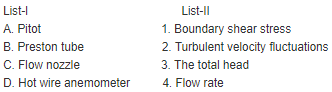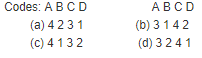Solution:

Ans. (b)

QUESTION: 19

Flow separation is caused by

Solution:

Ans. (c) Separation takes place where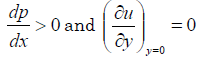QUESTION: 20

Flow separation is likely to take place when the pressure gradient inthe direction of flow is:

Solution:

Ans. (b)

QUESTION: 21

Flow separation is caused by:

Solution:

Ans. (c) i.e. an adverse pressure gradient.
When the pressure goes increasing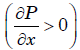in the direction of flow, the pressure force acts against the direction of direction of flow thus retarding the flow. This has an effect of retarding the flow in the boundary layer and hence thickenings the boundary layer more rapidly. This and the boundary shear bring the fluid in the boundary layer to rest and causes back flow. Due to this
the boundary layer no more sticks to the boundary but is shifted away from the
boundary. This phenomenon is called as “Boundary Layer Separation”.

QUESTION: 22

The ratio of the thickness of thermal boundary layer to the thickness of hydrodynamic boundary layer is equal to (Prandtl number)n, where nis:

Solution:

Ans. (a)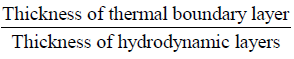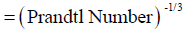QUESTION: 23

Hydrodynamic and thermal boundary layer thickness is equal forPrandtl number

Solution:

Ans. (c)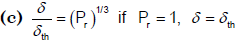QUESTION: 24

Which non-dimensional number relates the thermal boundary layerand hydrodynamic boundary layer?

Solution:

Ans. (d) Prandtl number relates the thermal boundary layer and hydrodynamic boundary layer.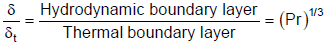QUESTION: 25

Consider the following statements pertaining to boundary layer:
1. Boundary layer is a thin layer adjacent to the boundary where maximum viscous energy dissipation takes place.
2. Boundary layer thickness is a thickness by which the ideal flow isshifted.
3. Separation of boundary layer is caused by presence of adverse pressure gradient.
Which of these statements are correct?

Solution:

Ans. (c) 2 is wrong it defines displacement thickness.
Boundary layer thickness: The thickness of the boundary layer is arbitrarily defined as that distance from the boundary in which the velocity reaches 99 percent of the velocity of the free stream. It is denoted by the symbol δ
Displacement thickness: dy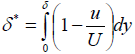It is the distance, measured perpendicular to the boundary, by which the main/free stream is displaced on account of formation of boundary layer.
or,
It is an additional "wall thickness" that would have to be added to compensate
for the reduction in flow rate on account of boundary layer formation

QUESTION: 26

In a convective heat transfer situation Reynolds number is very largebut the Prandtl number is so small that the product Re × Pr is less thanone in such a condition which one of the following is correct?

Solution:

Ans. (c)QUESTION: 27

Which one of the following is the correct relationship between the boundary layer thickness δ, displacement thickness δ* and the momentum thickness θ?

Solution:

Ans. (a) δ >δ*>θ >δ**

QUESTION: 28

For linear distribution of velocity in the boundary layer on a flat plate,what is the ratio of displacement thickness (δ*) to the boundary layer thickness (δ)?

Solution:

Ans. (c) Remember it.

QUESTION: 29

A flat plate, 2m × 0.4m is set parallel to a uniform stream of air (density1.2kg/m3 and viscosity 16 centistokes) with its shorter edges along theflow. The air velocity is 30 km/h. What is the approximate estimated thickness of boundary layer at the down stream end of the plate?

Solution:

Ans. (b) Thickness of Boundary layer,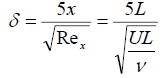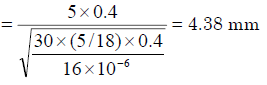QUESTION: 30

The ‘velocity defect law’ is so named because it governs a

Solution:

Ans. (c) Figure shows the logarithmic
velocity profile a little away from the wall. Velocity difference (Vmax – V) is known as velocity defect. So velocity defect law occurs due to occurrence of flow with a
logarithmic velocity profile a little away from the wall.